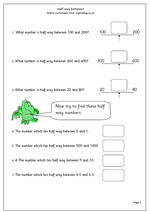# Year 4 Maths number worksheet: Half way betweenFinding the number which lies half way between two other numbers can be easy or quite tricky, even when using fairly small numbers. When using whole tens or hundreds the questions tend to be quite straightforward, as does finding halfway between two consecutive whole numbers. Most of the questions on this number worksheet are of this easier type. One of the harder questions here is to find half way between 20 and 80 and it is interesting to ask how people go about this mentally. Often they will conduct a mental process of adding ten to the lower number and subtracting ten from the higher number until they meet ‘in the middle’.